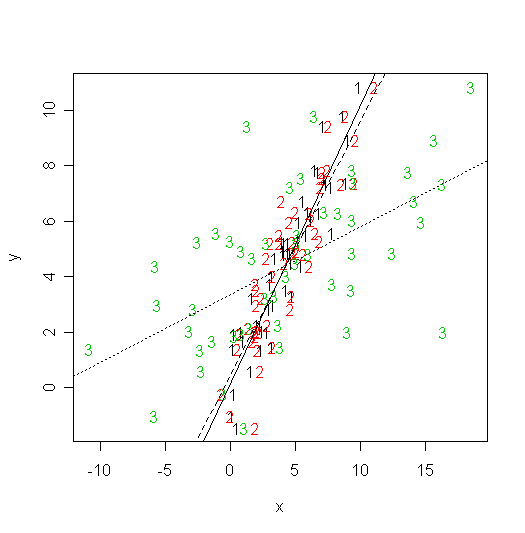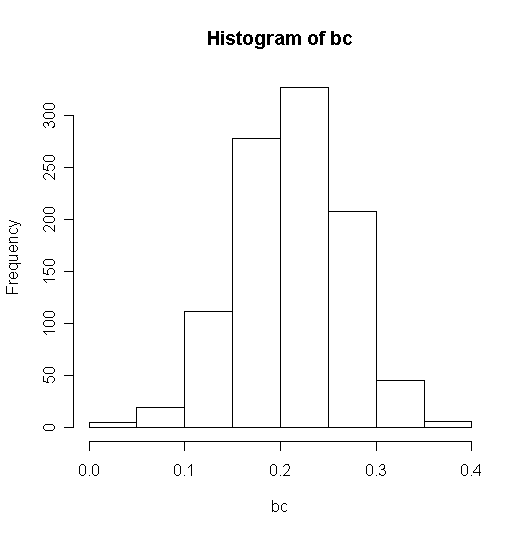# Errors in predictors (Reading: Faraway (2005, 1st edition), 5.1)

With real data, you never know the magnitude of the effect of errors in the predictors since we don't observe the true values. In this section we will simulate some data (so we know the true values of the parameters) and then add errors to the predictor to see the effect. First we generate some (xi, yi) pairs and then add increasing amounts of error to x. Remember the error is random so you won't get the exact same results if you repeat this experiment.

> x <- 10*runif(50) # x~U(0,10), 50 uniform random numbers
> y <- x+rnorm(50) #
b0=0, b1=1, e~U(0,1)
> m1 <- lm(y~x) # fit a simple linear regression model

> summary(m1) # should see estimates close to true values
Call:

lm(formula = y ~ x)

Residuals:

Min       1Q   Median       3Q      Max

-2.39959 -0.64664  0.01070  0.77001  2.20880

Coefficients:

Estimate Std. Error t value Pr(>|t|)

(Intercept)  0.13929    0.28620   0.487    0.629

x            1.00315    0.05738  17.484   <2e-16 ***

---

Signif. codes:  0 '***' 0.001 '**' 0.01 '*' 0.05 '.' 0.1 ' ' 1

Residual standard error: 1.036 on 48 degrees of freedom

Multiple R-Squared: 0.8643,     Adjusted R-squared: 0.8615

F-statistic: 305.7 on 1 and 48 DF,  p-value: < 2.2e-16

> z <- x+rnorm(50) # now add some standard normal error to x
> m2 <- lm(y~z)
> summary(m2)
# what happened to the estimates and the other quantities?

Call:

lm(formula = y ~ z)

Residuals:

Min       1Q   Median       3Q      Max

-3.68212 -0.84355  0.08807  0.78287  2.70036

Coefficients:

Estimate Std. Error t value Pr(>|t|)

(Intercept)  0.46071    0.34165   1.348    0.184

z            0.91511    0.06687  13.685   <2e-16 ***

---

Signif. codes:  0 '***' 0.001 '**' 0.01 '*' 0.05 '.' 0.1 ' ' 1

Residual standard error: 1.27 on 48 degrees of freedom

Multiple R-Squared: 0.796,      Adjusted R-squared: 0.7917

F-statistic: 187.3 on 1 and 48 DF,  p-value: < 2.2e-16

> z1 <- x+5*rnorm(50) # add even more error
> m3 <- lm(y~z1)
> summary(m3) # relationship between x and y is almost washed out

Call:

lm(formula = y ~ z1)

Residuals:

Min       1Q   Median       3Q      Max

-5.34926 -1.54357 -0.01277  1.32366  5.75679

Coefficients:

Estimate Std. Error t value Pr(>|t|)

(Intercept)   3.3447     0.3924   8.524 3.60e-11 ***

z1            0.2443     0.0495   4.935 1.01e-05 ***

---

Signif. codes:  0 '***' 0.001 '**' 0.01 '*' 0.05 '.' 0.1 ' ' 1

Residual standard error: 2.291 on 48 degrees of freedom

Multiple R-Squared: 0.3366,     Adjusted R-squared: 0.3228

F-statistic: 24.35 on 1 and 48 DF,  p-value: 1.006e-05

We can plot all this information:

> matplot(cbind(x,z,z1),y,xlab="x",ylab="y")
> abline(m1,lty=1)

> abline(m2,lty=2)

> abline(m3,lty=3)Note the slopes decrease when the errors in x have larger variance.

To get an idea of average behavior we need to repeat the experiment (I will do it 1000 times here). The slopes from the 1000 experiments are saved in vector bc:

> bc <- rep(0, 1000)

> for (i in 1:1000) {
+ y <- x + rnorm(50)
+ z <- x + 5*rnorm(50)
+ g <- lm(y~z)
+ bc[i] <- g\$coef
+ }
> summary(bc)

Min. 1st Qu.  Median    Mean 3rd Qu.    Max.

0.02461 0.17470 0.21470 0.21220 0.25110 0.38110

> hist(bc)Given that the variance of a standard uniform random variable is 1/12, sd2=25 and sx2=100/12, we'd expect the mean to be 0.25.